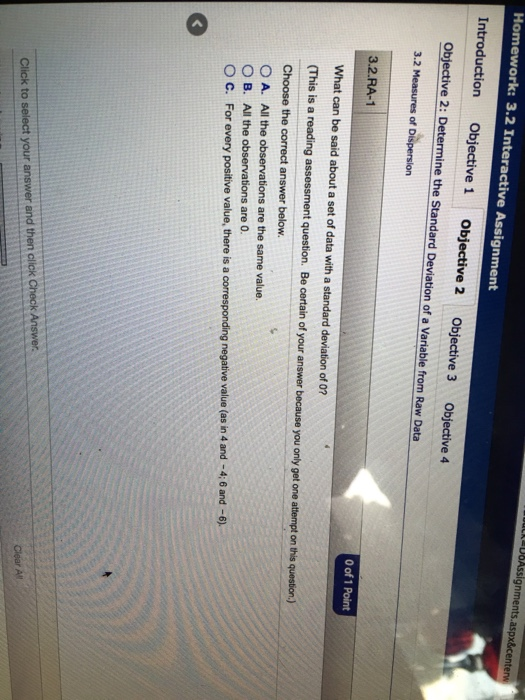# What can be said about a set of data with a standard deviation of 0?What can be said about a set of data with a standard deviation of 0? (This is a reading assessment question. Be certain of your answer because you only get one attempt on the question) Choose the correct answer below All the observations are the same value All the observations are 0 For every positive value, there is a corresponding negative value (as in 4 and -4. 6 and -6).

General guidance

Concepts and reason

Standard deviation:
The variation between each observation from its mean is known as standard deviation. The greater the distance of the points from the mean, the greater is the variability and vice-versa.

Fundamentals

Properties of standard deviation:
• Standard deviation is used to measure the spread of the data set.
• Value of standard deviation cannot be negative.
• Standard deviation is sensitive to the outliers.
• Standard deviation is measure of variability.
• The value of standard deviation is zero, if the all the observations in the data set are equal.

Step-by-step

Step 1 of 2

If the data set contains every positive value and corresponding negative value, then mean will be zero but not standard deviation because it represents square mean difference. Hence, the option C is wrong. Moreover, if all the values are 0, then standard deviation cannot be calculated for this type of data. Hence, the option B is wrong.

The incorrect options are identified by analyzing when the sum of the deviations between the mean and the observed value cannot be zero.

Use the properties of standard deviation to identify the correct option.

Step 2 of 2

If the all values in the data set are equal, then mean is equal to the data value. Then, the squared difference between every data value and mean value becomes zero. Hence, the standard deviation is zero.

All the observations are the same value.

The correct option is obtained by analyzing when the sum of the deviations between the mean and the observed value becomes zero.